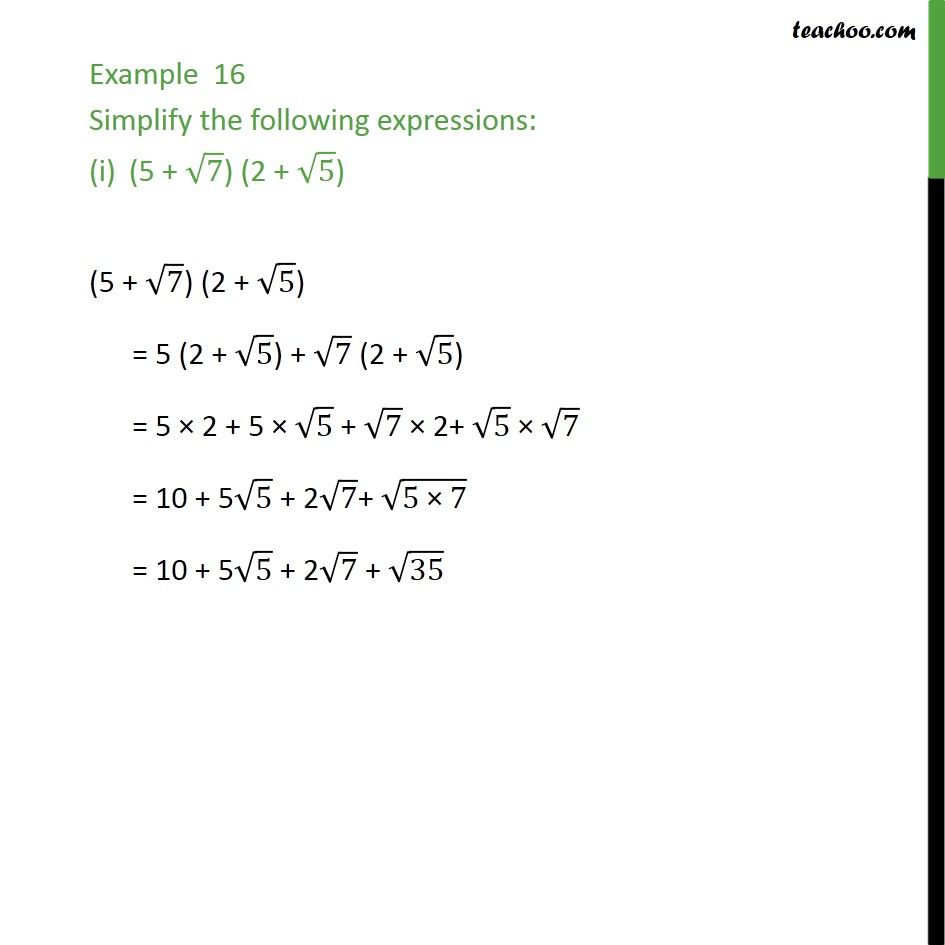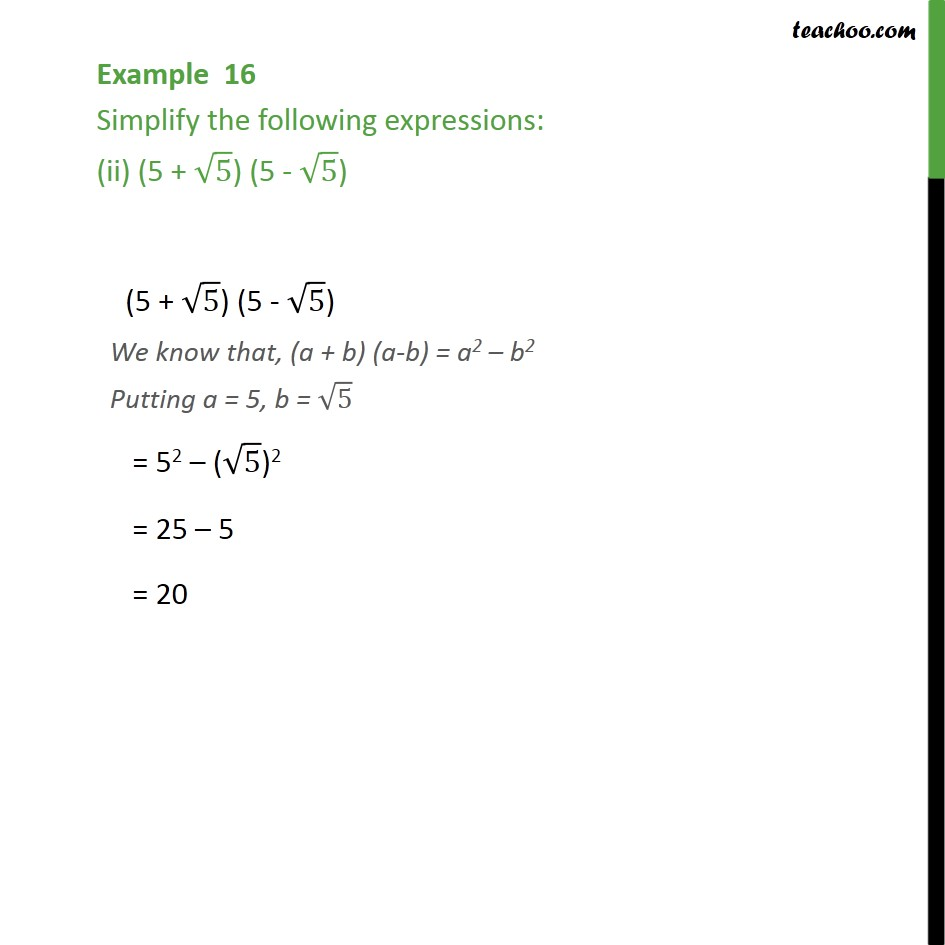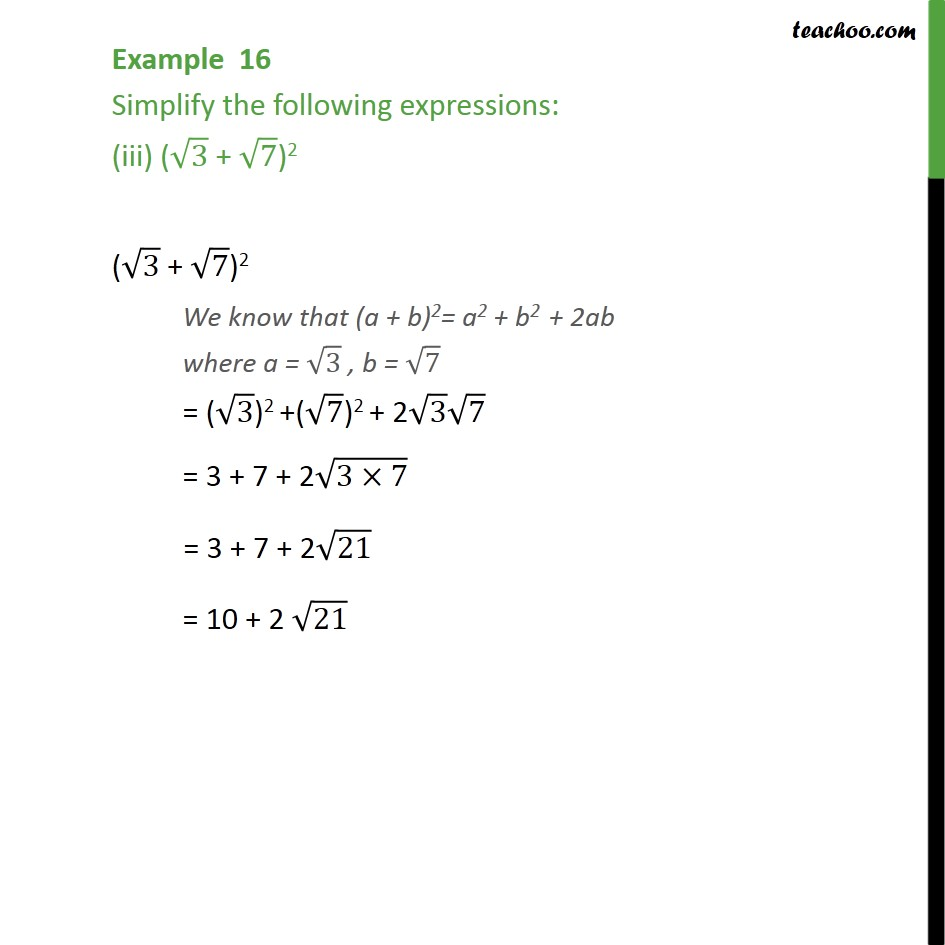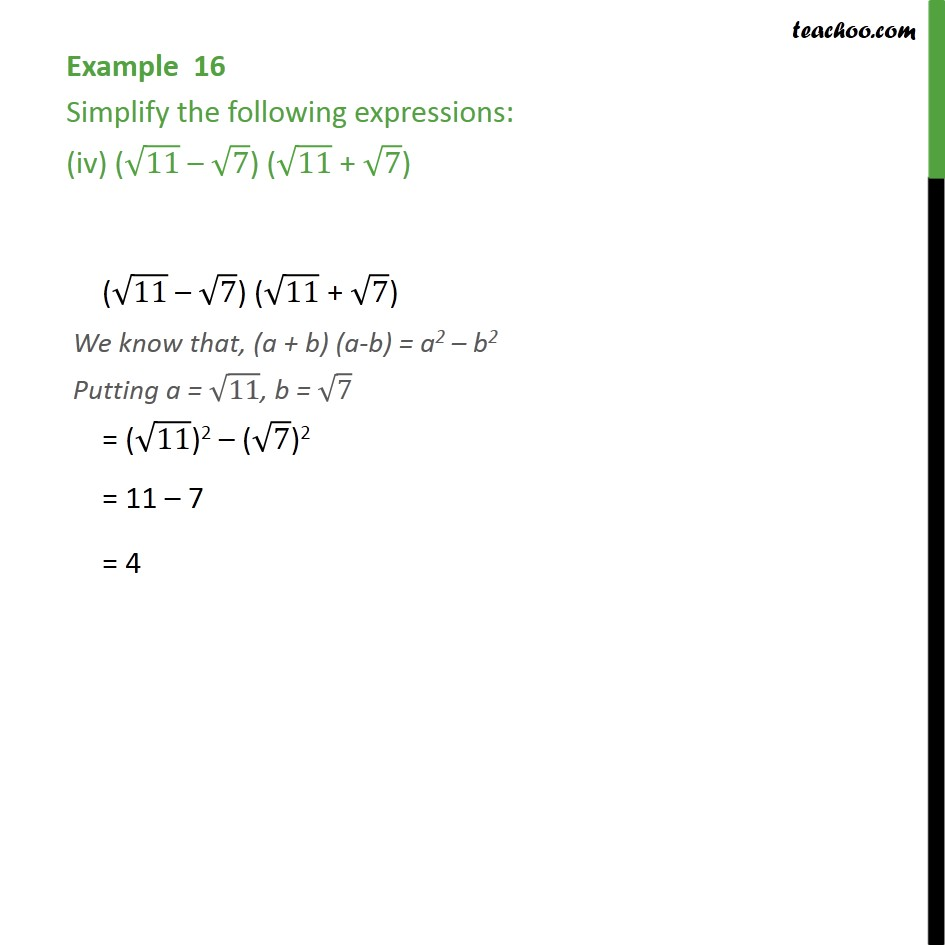1. Chapter 1 Class 9 Number Systems
2. Serial order wise
3. Examples

Transcript

Example 16 Simplify the following expressions: (5 + 7) (2 + 5) (5 + 7) (2 + 5) = 5 (2 + 5) + 7 (2 + 5) = 5 2 + 5 5 + 7 2+ 5 7 = 10 + 5 5 + 2 7+ (5" " 7) = 10 + 5 5 + 2 7 + 35 Example 16 Simplify the following expressions: (ii) (5 + 5) (5 - 5) (5 + 5) (5 - 5) We know that, (a + b) (a-b) = a2 b2 Putting a = 5, b = 5 = 52 ( 5)2 = 25 5 = 20 Example 16 Simplify the following expressions: (iii) ( 3 + 7)2 ( 3 + 7)2 We know that (a + b)2= a2 + b2 + 2ab where a = 3 , b = 7 = ( 3)2 +( 7)2 + 2 3 7 = 3 + 7 + 2 (3 7) = 3 + 7 + 2 21 = 10 + 2 21 Example 16 Simplify the following expressions: (iv) ( 11 7) ( 11 + 7) ( 11 7) ( 11 + 7) We know that, (a + b) (a-b) = a2 b2 Putting a = 11, b = 7 = ( 11)2 ( 7)2 = 11 7 = 4

Examples

About the AuthorDavneet Singh
Davneet Singh is a graduate from Indian Institute of Technology, Kanpur. He has been teaching from the past 10 years. He provides courses for Maths and Science at Teachoo.